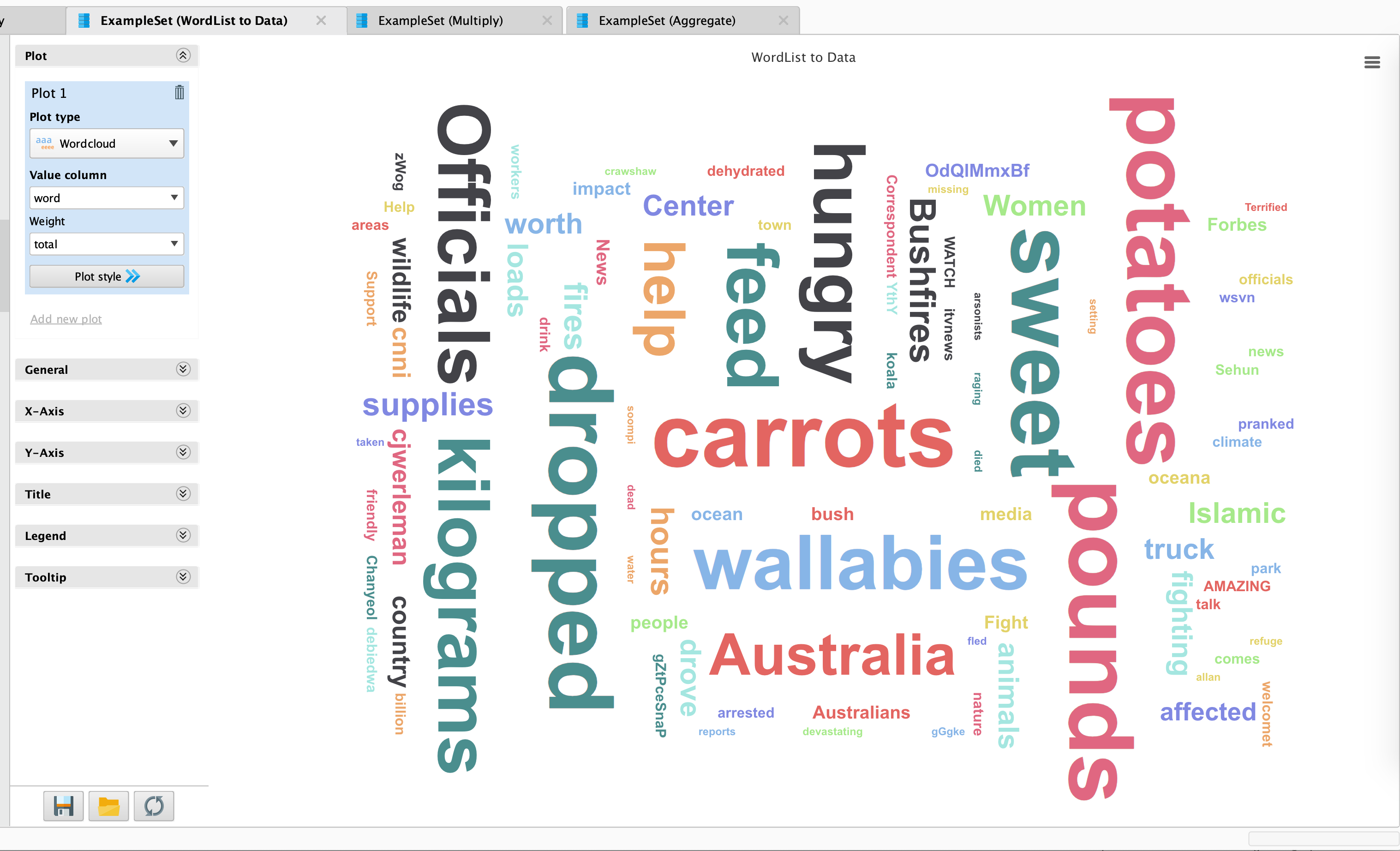Member Posts: 4Contributor I
Does anyone have an end to end Twitter Sentiment Analysis offering? I'm struggling with the piecemeal and fragmented responses on the forum and in the documentation.

By way of example, is it possible to mine twitter on say "Australian Bushfires" and:
a) produce a plot of the number of tweets by day over the past month,
b) produce a sentiment analysis and word cloud,
c) list and map the location of users.

There are many easy to follow guides for R. I'd like to do this using rapidminer.
Tagged:

• 12Administrator, Moderator, Employee, RapidMiner Certified Analyst, Community Manager, Member, University Professor, PM Moderator Posts: 2,717Community Manager
hi @DataEdLinks hmm doesn't sound too hard. Let me see if I can work a prototype for you.

Scott
• 12Administrator, Moderator, Employee, RapidMiner Certified Analyst, Community Manager, Member, University Professor, PM Moderator Posts: 2,717Community Manager
ok here's you go for (a) and (b):

```<?xml version="1.0" encoding="UTF-8"?><process version="9.5.001">
<context>
<input/>
<output/>
<macros/>
</context>
<operator activated="true" class="process" compatibility="9.5.001" expanded="true" name="Process">
<parameter key="logverbosity" value="init"/>
<parameter key="random_seed" value="-1"/>
<parameter key="send_mail" value="never"/>
<parameter key="process_duration_for_mail" value="30"/>
<parameter key="encoding" value="SYSTEM"/>
<process expanded="true">
<operator activated="false" class="retrieve" compatibility="9.5.001" expanded="true" height="68" name="Retrieve Twitter" width="90" x="45" y="85">
</operator>
<parameter key="connection_source" value="repository"/>
<parameter key="query" value="Australian Bushfires"/>
<parameter key="result_type" value="recent or popular"/>
<parameter key="limit" value="100"/>
<parameter key="filter_by_geo_location" value="false"/>
</operator>
<operator activated="true" class="retrieve" compatibility="9.5.001" expanded="true" height="68" name="Retrieve Twitter for Gary - search results" width="90" x="45" y="289">
<parameter key="repository_entry" value="Twitter for Gary - search results"/>
</operator>
<operator activated="true" class="multiply" compatibility="9.5.001" expanded="true" height="124" name="Multiply" width="90" x="313" y="187"/>
<operator activated="true" class="subprocess" compatibility="9.5.001" expanded="true" height="82" name="Subprocess (2)" width="90" x="514" y="493">
<process expanded="true">
<operator activated="true" class="nominal_to_text" compatibility="9.5.001" expanded="true" height="82" name="Nominal to Text" width="90" x="45" y="34">
<parameter key="attribute_filter_type" value="single"/>
<parameter key="attribute" value="Text"/>
<parameter key="attributes" value=""/>
<parameter key="use_except_expression" value="false"/>
<parameter key="value_type" value="nominal"/>
<parameter key="use_value_type_exception" value="false"/>
<parameter key="except_value_type" value="file_path"/>
<parameter key="block_type" value="single_value"/>
<parameter key="use_block_type_exception" value="false"/>
<parameter key="except_block_type" value="single_value"/>
<parameter key="invert_selection" value="false"/>
<parameter key="include_special_attributes" value="false"/>
</operator>
<operator activated="true" class="text:process_document_from_data" compatibility="8.2.000" expanded="true" height="82" name="Process Documents from Data" width="90" x="179" y="34">
<parameter key="create_word_vector" value="true"/>
<parameter key="vector_creation" value="Binary Term Occurrences"/>
<parameter key="keep_text" value="false"/>
<parameter key="prune_method" value="percentual"/>
<parameter key="prune_below_percent" value="3.0"/>
<parameter key="prune_above_percent" value="30.0"/>
<parameter key="prune_below_rank" value="0.05"/>
<parameter key="prune_above_rank" value="0.95"/>
<parameter key="datamanagement" value="double_sparse_array"/>
<parameter key="data_management" value="auto"/>
<parameter key="select_attributes_and_weights" value="false"/>
<list key="specify_weights"/>
<process expanded="true">
<operator activated="true" class="text:tokenize" compatibility="8.2.000" expanded="true" height="68" name="Tokenize" width="90" x="45" y="34">
<parameter key="mode" value="non letters"/>
<parameter key="characters" value=".:"/>
<parameter key="language" value="English"/>
<parameter key="max_token_length" value="3"/>
</operator>
<operator activated="true" class="text:filter_stopwords_english" compatibility="8.2.000" expanded="true" height="68" name="Filter Stopwords (English)" width="90" x="179" y="34"/>
<operator activated="true" class="text:filter_by_length" compatibility="8.2.000" expanded="true" height="68" name="Filter Tokens (by Length)" width="90" x="313" y="34">
<parameter key="min_chars" value="4"/>
<parameter key="max_chars" value="25"/>
</operator>
<connect from_port="document" to_op="Tokenize" to_port="document"/>
<connect from_op="Tokenize" from_port="document" to_op="Filter Stopwords (English)" to_port="document"/>
<connect from_op="Filter Stopwords (English)" from_port="document" to_op="Filter Tokens (by Length)" to_port="document"/>
<connect from_op="Filter Tokens (by Length)" from_port="document" to_port="document 1"/>
<portSpacing port="source_document" spacing="0"/>
<portSpacing port="sink_document 1" spacing="0"/>
<portSpacing port="sink_document 2" spacing="0"/>
</process>
</operator>
<operator activated="true" class="text:wordlist_to_data" compatibility="8.2.000" expanded="true" height="82" name="WordList to Data" width="90" x="313" y="34"/>
<connect from_port="in 1" to_op="Nominal to Text" to_port="example set input"/>
<connect from_op="Nominal to Text" from_port="example set output" to_op="Process Documents from Data" to_port="example set"/>
<connect from_op="Process Documents from Data" from_port="word list" to_op="WordList to Data" to_port="word list"/>
<connect from_op="WordList to Data" from_port="example set" to_port="out 1"/>
<portSpacing port="source_in 1" spacing="0"/>
<portSpacing port="source_in 2" spacing="0"/>
<portSpacing port="sink_out 1" spacing="0"/>
<portSpacing port="sink_out 2" spacing="0"/>
</process>
<description align="center" color="transparent" colored="false" width="126">for wordcloud</description>
</operator>
<operator activated="true" class="operator_toolbox:extract_sentiment" compatibility="2.3.000" expanded="true" height="82" name="Extract Sentiment" width="90" x="514" y="289">
<parameter key="text_attribute" value="Text"/>
<parameter key="use_default_tokenization_regex" value="true"/>
</operator>
<operator activated="true" class="subprocess" compatibility="9.5.001" expanded="true" height="82" name="Subprocess" width="90" x="514" y="34">
<process expanded="true">
<operator activated="true" class="date_to_nominal" compatibility="9.5.001" expanded="true" height="82" name="Date to Nominal" width="90" x="45" y="34">
<parameter key="attribute_name" value="Created-At"/>
<parameter key="date_format" value="MM/dd/yyyy"/>
<parameter key="time_zone" value="America/New_York"/>
<parameter key="locale" value="English (United States)"/>
<parameter key="keep_old_attribute" value="false"/>
</operator>
<operator activated="true" class="nominal_to_date" compatibility="9.5.001" expanded="true" height="82" name="Nominal to Date" width="90" x="179" y="34">
<parameter key="attribute_name" value="Created-At"/>
<parameter key="date_type" value="date"/>
<parameter key="date_format" value="MM/dd/yyyy"/>
<parameter key="time_zone" value="America/New_York"/>
<parameter key="locale" value="English (United States)"/>
<parameter key="keep_old_attribute" value="false"/>
</operator>
<connect from_port="in 1" to_op="Date to Nominal" to_port="example set input"/>
<connect from_op="Date to Nominal" from_port="example set output" to_op="Nominal to Date" to_port="example set input"/>
<connect from_op="Nominal to Date" from_port="example set output" to_port="out 1"/>
<portSpacing port="source_in 1" spacing="0"/>
<portSpacing port="source_in 2" spacing="0"/>
<portSpacing port="sink_out 1" spacing="0"/>
<portSpacing port="sink_out 2" spacing="0"/>
</process>
<description align="center" color="transparent" colored="false" width="126">convert &amp;quot;Created-At&amp;quot; to date only</description>
</operator>
<operator activated="true" class="aggregate" compatibility="9.5.001" expanded="true" height="82" name="Aggregate" width="90" x="648" y="34">
<parameter key="use_default_aggregation" value="false"/>
<parameter key="attribute_filter_type" value="all"/>
<parameter key="attribute" value=""/>
<parameter key="attributes" value=""/>
<parameter key="use_except_expression" value="false"/>
<parameter key="value_type" value="attribute_value"/>
<parameter key="use_value_type_exception" value="false"/>
<parameter key="except_value_type" value="time"/>
<parameter key="block_type" value="attribute_block"/>
<parameter key="use_block_type_exception" value="false"/>
<parameter key="except_block_type" value="value_matrix_row_start"/>
<parameter key="invert_selection" value="false"/>
<parameter key="include_special_attributes" value="false"/>
<parameter key="default_aggregation_function" value="average"/>
<list key="aggregation_attributes">
<parameter key="Created-At" value="count"/>
</list>
<parameter key="group_by_attributes" value="Created-At"/>
<parameter key="count_all_combinations" value="false"/>
<parameter key="only_distinct" value="false"/>
<parameter key="ignore_missings" value="true"/>
<description align="center" color="transparent" colored="false" width="126">count tweets by date</description>
</operator>
<connect from_op="Retrieve Twitter for Gary - search results" from_port="output" to_op="Multiply" to_port="input"/>
<connect from_op="Multiply" from_port="output 1" to_op="Subprocess" to_port="in 1"/>
<connect from_op="Multiply" from_port="output 2" to_op="Extract Sentiment" to_port="exa"/>
<connect from_op="Multiply" from_port="output 3" to_op="Subprocess (2)" to_port="in 1"/>
<connect from_op="Subprocess (2)" from_port="out 1" to_port="result 3"/>
<connect from_op="Extract Sentiment" from_port="exa" to_port="result 2"/>
<connect from_op="Subprocess" from_port="out 1" to_op="Aggregate" to_port="example set input"/>
<connect from_op="Aggregate" from_port="example set output" to_port="result 1"/>
<portSpacing port="source_input 1" spacing="0"/>
<portSpacing port="sink_result 1" spacing="0"/>
<portSpacing port="sink_result 2" spacing="252"/>
<portSpacing port="sink_result 3" spacing="126"/>
<portSpacing port="sink_result 4" spacing="21"/>
<description align="center" color="yellow" colored="false" height="217" resized="true" width="173" x="16" y="14">You will need you use your own Twitter Connection here</description>
<description align="center" color="yellow" colored="false" height="206" resized="false" width="211" x="464" y="437">You will need Text Processing for this</description>
<description align="center" color="yellow" colored="false" height="206" resized="false" width="211" x="465" y="222">You will need Operator Toolbox for this</description>
</process>
</operator>
</process>
```

Word cloud looks like this:(c) is tricky as Twitter seems to be suppressing the geo-location fields from what I can see. See attached ExampleSet that I just did using "Australian Bushfires". If you have Twitter results with the geocoding, then it's just another visualization.

Note that the visualizations you ask for you are not done in a process but rather in the Results view. Word Clouds (for (b) )and line graphs (for (a) ) are done this way.

Scott